# Test: Introduction to Quadratic Equations

Test Description

## 30 Questions MCQ Test NCERT Mathematics for CAT Preparation | Test: Introduction to Quadratic Equations

Test: Introduction to Quadratic Equations for Class 10 2022 is part of NCERT Mathematics for CAT Preparation preparation. The Test: Introduction to Quadratic Equations questions and answers have been prepared according to the Class 10 exam syllabus.The Test: Introduction to Quadratic Equations MCQs are made for Class 10 2022 Exam. Find important definitions, questions, notes, meanings, examples, exercises, MCQs and online tests for Test: Introduction to Quadratic Equations below.
Solutions of Test: Introduction to Quadratic Equations questions in English are available as part of our NCERT Mathematics for CAT Preparation for Class 10 & Test: Introduction to Quadratic Equations solutions in Hindi for NCERT Mathematics for CAT Preparation course. Download more important topics, notes, lectures and mock test series for Class 10 Exam by signing up for free. Attempt Test: Introduction to Quadratic Equations | 30 questions in 30 minutes | Mock test for Class 10 preparation | Free important questions MCQ to study NCERT Mathematics for CAT Preparation for Class 10 Exam | Download free PDF with solutions
 1 Crore+ students have signed up on EduRev. Have you?
Test: Introduction to Quadratic Equations - Question 1

### Write the general form of a quadratic polynomia

Detailed Solution for Test: Introduction to Quadratic Equations - Question 1

If the coefficient of x2 is zero , then the equation is not a quadratic equation , its a linear equation. So its necessary condition for the quadratic equation.

Test: Introduction to Quadratic Equations - Question 2

### One of the roots of the quadratic equation 6x2 – x – 2 = 0 is:​

Test: Introduction to Quadratic Equations - Question 3

### Which of the following statement is TRUE?

Detailed Solution for Test: Introduction to Quadratic Equations - Question 3

A quadratic equation in variable x is of the form ax2+ bx + c = 0, where a, b, c are real numbers a ≠ o, because if a=0 then the equation becomes a linear equation.
If we can factorise ax2 + bx + c, a ≠ 0 into product of two linear factors then roots can be found by equating each factor to zero because if two factors are in multiplication and equal to zero then either of the factor is zero.
A real number R is said to be a root of the quadratic equation ax2 + bx + c = 0 if a(R)2 + bR + c = 0. , root means that the value gives answer equal to zero.
So all are correct.

Test: Introduction to Quadratic Equations - Question 4

If n is a non negative integer, then ann +…+ a 1 x + a0 is a

Test: Introduction to Quadratic Equations - Question 5

The two positive numbers differ by 5 and square of their sum is 169 are

Test: Introduction to Quadratic Equations - Question 6

If 4 is a root of the equation x2 + 3x + k = 0, then k is​

Detailed Solution for Test: Introduction to Quadratic Equations - Question 6

4 is the solution , this means that if we put x=4 we get 0. So putting x=4 in the equation x2+3x+k=0 we get 42+3*4+k=0
16+12+k=0 ⇒ k=-28

Test: Introduction to Quadratic Equations - Question 7

If 8 is a root of the equation x2 – 10x + k = 0, then the value of k is:​

Detailed Solution for Test: Introduction to Quadratic Equations - Question 7

Let, p(x) = x²-10x+k
since, 8 is the root of p(x)
∴ p(8) = 0
8²-10(8)+k = 0
64-80+k = 0
-16+k = 0
⇒ k = 16 .

Test: Introduction to Quadratic Equations - Question 8

Which of following is not a quadratic equation:

Test: Introduction to Quadratic Equations - Question 9

The solution of 5z2 = 3z is​

Detailed Solution for Test: Introduction to Quadratic Equations - Question 9

We have 5z2=3z
5z2-3z=0
z(5z-3)=0
So either z=0
Or 5z-3 =0  = z=⅗. So there are two solutions

Test: Introduction to Quadratic Equations - Question 10

Which of the following equations has 2 as a root?

Test: Introduction to Quadratic Equations - Question 11

Which of the following equations has the sum of its roots as 3?

*Multiple options can be correct
Test: Introduction to Quadratic Equations - Question 12

Which of the following is not a quadratic equation:​

Detailed Solution for Test: Introduction to Quadratic Equations - Question 12

Option (B) and (D) , both are the correct answers.  We have x(x + 1) + 8 = (x + 2) (x – 2)
=x+ x + 8 = x2 - 4
= x = -12, which is not a quadratic equation
Also, in (B) (x + 2)2 = x3 – 4
=x+4x + 4=x- 4, which is a cubic equation

Test: Introduction to Quadratic Equations - Question 13

If x = -2 is a root of equation x2 – 4x + K = 0 then value of K is

Test: Introduction to Quadratic Equations - Question 14

The same value of x satisfies the equations 4x + 5 = 0 and 4x2 + (5 + 3p)x + 3p= 0, then p is​

Test: Introduction to Quadratic Equations - Question 15

If x = 1 is a root of equation x2 – Kx + 5 = 0 then value of K is

Detailed Solution for Test: Introduction to Quadratic Equations - Question 15

Root of the equation means that the value when substituted in the equation gives zero as answer.
x- kx + 5 = 0
Putting x = 1
1*1 -k + 5 = 0
-k+6=0
k=6

Test: Introduction to Quadratic Equations - Question 16

The equation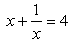in standard form ax2 + bx + c = 0 is written as :

Detailed Solution for Test: Introduction to Quadratic Equations - Question 16

We have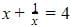Taking LCM,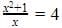Multiplying LHS and RHS by x
x2+1=4x
x2-4x+1=0
Which is the required equation.

Test: Introduction to Quadratic Equations - Question 17

If x = 1 is a common root of the equation x2 + ax – 3 = 0 and bx2 – 7x + 2 = 0 then ab =

Test: Introduction to Quadratic Equations - Question 18

If the area of a rectangle is 24 m2 and its perimeter is 20 m, the equation to find its length and breadth would be:​

Test: Introduction to Quadratic Equations - Question 19

If x = 2 is a root of equation x2 + 3x – k = 0 then value of k is

Test: Introduction to Quadratic Equations - Question 20

The roots of the equation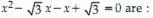Detailed Solution for Test: Introduction to Quadratic Equations - Question 20

x- √3x - x + √3 = 0
Taking common x from first two terms
x (x - √3) - 1 (x - √3) = 0
(x - √3) (x - 1) = 0
So, either x = √3 or x = 1

Test: Introduction to Quadratic Equations - Question 21

The condition for equation ax2 + bx + c = 0 to be quadratic is​

Detailed Solution for Test: Introduction to Quadratic Equations - Question 21

For ax+ bx + c = 0 to be a quadratic equation a0because if a is zero then ax= 0 So we are left with only bx + c = 0 which is a linear equation in one variable. So to be a quadratic equation ax2 cannot be zero.

Test: Introduction to Quadratic Equations - Question 22

The value of q if x = 2 is a solution of 8x2 + qx – 4 = 0 is _____​

Test: Introduction to Quadratic Equations - Question 23

The equation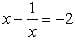in standard form ax2 + bx + c = 0 is written as

Test: Introduction to Quadratic Equations - Question 24

Zeroes of the quadratic polynomial ax2 + bx + c and roots of the quadratic equation ax2 + bx + c = 0 are ——–​

Detailed Solution for Test: Introduction to Quadratic Equations - Question 24

Zeros of the polynomial means the value of variable such that the equation is equal to zero.Roots of the equation means the value of the variable for which LHS=RHS which basically means that the equation is equal to zero. Hence Zeros and roots are one and the same thing.

Test: Introduction to Quadratic Equations - Question 25

The condition for equation ax2 + bx + c = 0 to be linear is​

Test: Introduction to Quadratic Equations - Question 26

The solution of x2 + 4x + 4 = 0 is​

Test: Introduction to Quadratic Equations - Question 27

If x2 + 2 kx + 4 = 0 has a root x = 2, then the value of k is?

Test: Introduction to Quadratic Equations - Question 28

Solve 9x2 = 36​

Test: Introduction to Quadratic Equations - Question 29

The value/s of x when (x – 4) (3x + 2) = 0 ________​

Test: Introduction to Quadratic Equations - Question 30

The positive root of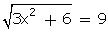is

## NCERT Mathematics for CAT Preparation

276 docs|149 tests
 Use Code STAYHOME200 and get INR 200 additional OFF Use Coupon Code